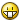## Recommended Posts

Actually it is 'just' from base 2 to base 36 (all digits and all letters)... But it should be enough in most cases.

```MsgBox(0, '', _Any2Dec('ff', 16))

MsgBox(0, '', _Dec2Any('127', 2))

#region;_Any2Dec, _Dec2Any
Func _Any2Dec(\$sNum, \$iBase)
If \$iBase < 2 Or \$iBase > 36 Then Return 0

\$sNum = StringReplace(\$sNum, ' ', '')
Local \$iSep, \$fOutPut = 0, \$iDigit, \$c, \$iL, \$bNeg = 0, \$sValidDigits

If StringLeft(\$sNum, 1) = '-' Then
\$bNeg = 1
\$sNum = StringTrimLeft(\$sNum, 1)
EndIf

If \$iBase <= 10 Then
For \$c = 0 to (\$iBase - 1)
\$sValidDigits = \$sValidDigits & '|' & \$c
Next
\$sValidDigits = StringTrimLeft(\$sValidDigits, 1)
Else
\$sValidDigits = '0|1|2|3|4|5|6|7|8|9'
For \$c = 10 To \$iBase - 1
\$sValidDigits = \$sValidDigits & '|' & Chr(55 + \$c)
Next
EndIf

If Not _Only(\$sNum, \$sValidDigits & '|.') Then
Return 0
EndIf

\$iSep = StringInStr(\$sNum, '.')
If \$iSep <> 0 Then
\$sNum = StringReplace(\$sNum, '.', '')
\$iSep = (StringLen(\$sNum) - \$iSep + 1) * - 1
EndIf

\$iL = StringLen(\$sNum)
For \$c = 1 To StringLen(\$sNum)
\$iDigit = StringMid(\$sNum, \$iL - \$c + 1, 1)
Select
Case Asc(\$iDigit) >= 97
\$iDigit = Asc(\$iDigit) - 87
CAse Asc(\$iDigit) >= 65
\$iDigit = Asc(\$iDigit) - 55
Case Else
\$iDigit = Int(\$iDigit)
EndSelect

\$fOutPut = \$fOutPut + \$iDigit * \$iBase^ (\$iSep + \$c - 1)
Next

If \$bNeg = 1 Then \$fOutPut = \$fOutPut * - 1
Return \$fOutPut
EndFunc;==>_Any2Dec

Func _Only(\$string, \$aItems)
\$aItems = StringSplit(\$aItems, '|')
Local \$bOk, \$c, \$c2, \$char

For \$c = 1 To StringLen(\$string)
\$char = StringMid(\$string, \$c, 1)
\$bOk = 0
For \$c2 = 1 To \$aItems
If \$aItems[\$c2] = \$char Then
\$bOk = 1
ExitLoop
EndIf
Next

If \$bOk = 0 Then
Return 0
EndIf
Next

Return 1
EndFunc;==>_Only

Func _Dec2Any(\$sNum, \$iBase)
If \$iBase < 2 Or \$iBase > 36 Then Return 0

\$sNum = StringReplace(\$sNum, ' ', '')
Local \$aParts, \$iDigit, \$sOutPut, \$bNeg = 0

If StringLeft(\$sNum, 1) = '-' Then
\$bNeg = 1
\$sNum = StringTrimLeft(\$sNum, 1)
EndIf

If StringInStr(\$sNum, '.') Then
\$aParts = StringSplit(\$sNum, '.')
\$aParts = Int(\$aParts)
\$aParts = \$aParts / 10 ^ StringLen(\$aParts)
Else
\$aParts = Int(\$sNum)
\$aParts = 0
EndIf

While \$aParts <> 0
\$iDigit = Mod(\$aParts, \$iBase)
If \$iDigit >= 10 Then
\$iDigit = Chr(55 + \$iDigit);65 is A
EndIf
\$sOutPut = \$iDigit & \$sOutPut
\$aParts = Int(\$aParts / \$iBase)
Wend
If \$aParts <> 0 Then
\$sOutPut = \$sOutPut & '.'
While \$aParts <> 0
\$aParts = \$aParts * \$iBase
\$iDigit = Int(\$aParts)
\$aParts = \$aParts - \$iDigit
If \$iDigit >= 10 Then
\$iDigit = Chr(55 + \$iDigit);65 is A
EndIf
\$sOutPut = \$sOutPut & \$iDigit
Wend
EndIf

If \$bNeg = 1 Then \$sOutPut = '-' & \$sOutPut
Return \$sOutPut
EndFunc;==>_Dec2Any
#endregion```

Edit: Sorry bug.... Delete the version you downloaded if you do and wait....Edit2: Fake alarm.It was just a little problem with lowecase letters... Anyway, updated version.

Edit3: Fixing the bug about lowercase I made one about uppercase... Oh dear. Should be final this time.

Edited by ezzetabi

##### Share on other sites

Nice. I'm gonna try it out.

Easier than using MS Calculator.

##### Share on other sites

and MS Calc can't convert non integers!

##### Share on other sites

Yesterday I used it to convert a display resolution.

And it worked fine.

I just tested Dec2Any with binary numbers and it works as expected.

Great job.

Did you make it from scratch?

##### Share on other sites

Yes, of course!

If I use other ones code I always write the name of the author.

## Create an account

Register a new account

• ### Recently Browsing   0 members

×

• Wiki

• Back

• #### Beta

• Git
• FAQ
• Our Picks
×
• Create New...